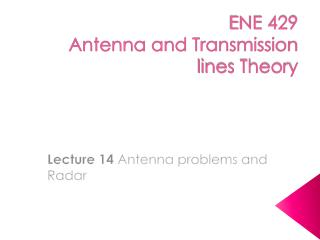Download PresentationENE 429 Antenna and Transmission lines Theory

# ENE 429 Antenna and Transmission lines Theory - PowerPoint PPT Presentation

ENE 429 Antenna and Transmission lines Theory. Lecture 14 Antenna problems and Radar. Review (1). Small loop antenna (magnetic dipole) Dipole antenna generates high radiation resistance and efficiency For far field region, where. Review (2). Half-wave dipole.I am the owner, or an agent authorized to act on behalf of the owner, of the copyrighted work described.
Download Presentation## ENE 429 Antenna and Transmission lines Theory

Download Policy: Content on the Website is provided to you AS IS for your information and personal use and may not be sold / licensed / shared on other websites without getting consent from its author.While downloading, if for some reason you are not able to download a presentation, the publisher may have deleted the file from their server.

- - - - - - - - - - - - - - - - - - - - - - - - - - E N D - - - - - - - - - - - - - - - - - - - - - - - - - -
Presentation Transcript
1. ENE 429Antenna and Transmission lines Theory Lecture 14Antenna problems and Radar

2. Review (1) • Small loop antenna (magnetic dipole) • Dipole antenna generates high radiation resistance and efficiency For far field region, where

3. Review (2) • Half-wave dipole p = 7.658, Dmax = 1.64, Rrad = 73.2 

4. Monopole antenna • Image theory is employed to build a quarter-wave monopole antenna.

5. Mirrored image charge of opposite polarity

6. Monopole antenna properties • Monopole antenna is excited by a current source at its base. • Directivity is doubled and radiation resistance is half of that of dipole antenna.

7. Practical considerations • The best operation: ground is highly conductive (or use counterpoise in case of remote antenna) • Shorter than /4 antenna arises highly capacitive input impedances, thus efficiency decreases. • Solution: inductive coil or top-hat capacitor Inductive coil Top-hat capacitor

8. Antenna arrays • A group of several antenna elements in various configurations (straight lines, circles, triangles, etc.) with proper amplitude and phase relations, main beam direction can be controlled. • Improvement of the radiation characteristic can be done over a single-element antenna (broad beam, low directivity)

9. Two-element arrays (1) To simplify, • All antennas are identical. • Current amplitude is the same. • The radiation pattern lies in x-y plane From Consider ,

10. Two-element arrays (2) Let I1 = I0, I2= I0ej, since r1and r2 >> d/2 for far field, we can assume 1  2  and r1  r2 r.

11. Two-element arrays (3) But the exponential terms cannot be approximated, then

12. Principle of pattern multiplication We can write this as Funit = a unit factor or the maximum time-averaged power density for an individual element at Farray = array factor = where This depends only on distance d and relative current phase, . We can conclude that the pattern function of an array of identical elements is described by the product of the element factor and the array factor.

13. N-element linear arrays We will simplify assumptions as follows: • The array is linear, evenly spaced along the line. • The array is uniform, driven by the same magnitude current source with constant phase difference between adjacent elements. (Farray)max = N2

14. Parasitic arrays • Yagiuda (rooftop antenna) Parasitic elements are indirectly driven by current induced in them from the driven element.

15. Friis transmission equation (1) • Consider power transmission relation between transmitting and receiving antennas where particular antennas are aligned with same polarization. Let Prad1be Ptotal radiated by antenna 1 have a directivity Dmax1, With reciprocal property, Therefore, we have

16. Friis transmission equation (2) Each variable is independent of one another, so each term has to be constant, we found that

17. Friis transmission equation (3) • Effective area (Ae)is much larger than the physical cross section. • More general expressions We can also write

18. Friis transmission equation (4) Finally, consider Prad = etPin, Pout = erPrec, and Gt = etDt, Gr = erDr Friis transmission equation Note: Assume - matched impedance condition between the transmitter circuitry/antenna and receiver - antenna polarizations are the same.

20. Receiver matching (2) • Since the receiver is matched, half the received power is dissipated in the load, therefore • Without the matching network,

21. Radar (Radio detection and ranging) • A monostatic radar system • Some of energy is scattered by target so called ‘the echo signal’ received at the radar antenna.

22. Radiated power Let Prad be the radiated power transmitted by the radar antenna, then the radiated power density P1(r, , ) at the target at the distance r away is The power scattered by the target is then s = radar cross section (m2)

23. Radar equation This scattered power results in a radiated power density at the radar antenna of Then By manipulation of these equations, we have or

24. More on antennas • Radiation patterns for dipole antenna http://www.amanogawa.com/archive/DipoleAnt/DipoleAnt-2.html

25. More examples Ex1 Suppose a 0.5 dipole transmitting antenna’s power source is 12-V amplitude voltage in series with a 25  source resistance as shown. What is the total power radiated from the antenna with and without an insertion of a matching network? 0.5

26. Ex2 Determine the array factor and normalized power function for five antenna elements spaced /4 apart with progressive phase steps of 30. The antennas are assumed to be a linear array of z-oriented dipole on the x-axis.

27. Ex3 Consider a pair of half-wavelength dipole antennas, separated by 1 km and aligned for maximum power transfer as shown. This transmission antenna is driven by 1 kW of power at 1 GHz. Assuming the antennas are 100% efficient, what is the receiving antenna’s output power Pout?